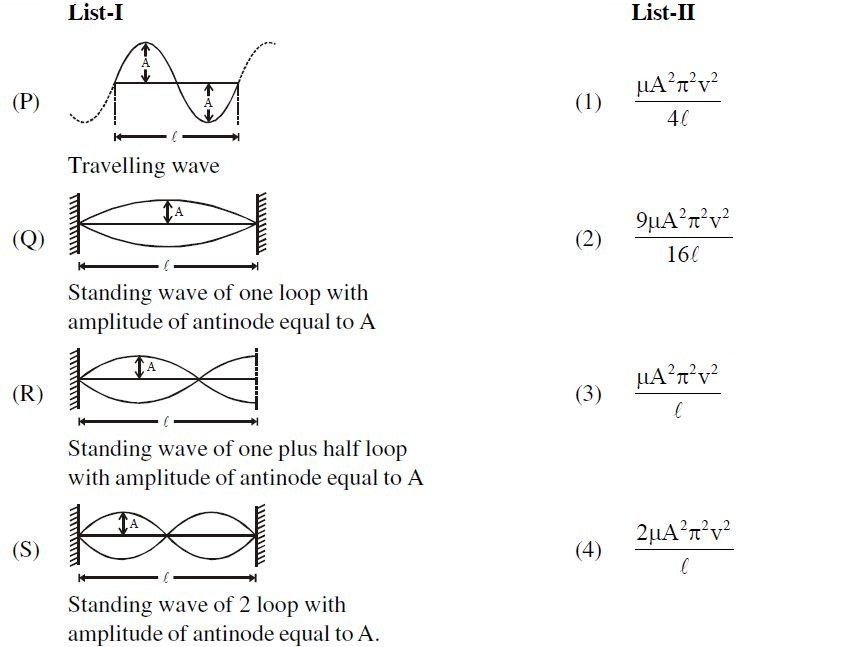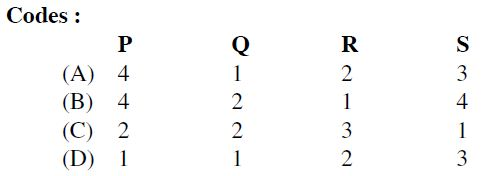# Energy of Waves on String!

List-$I$ shows four system of string each of same length, for producing travelling wave or standing wave. In each case velocity of travelling wave on string is same i.e.$v$. Match each system of list-$I$ with expressions given in list-$II$ describing the energy of the wave.×